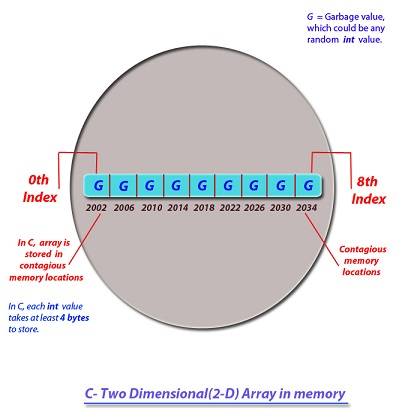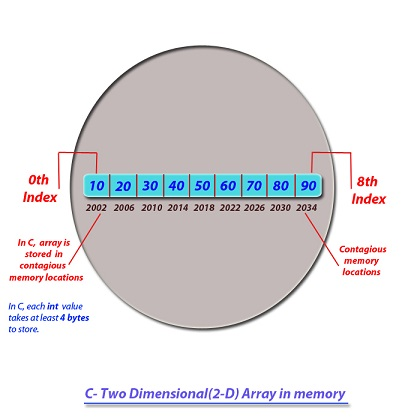Next >

# C - two-dimensional Array

A two-dimensional(2D) array is used to contain multiple arrays, which are holding the values of same type. The elements of a 2D array are arranged in rows and columns. A two-dimensional array contains arrays that are holding primitive values of the same type.

We will understand a two-dimensional(2D)array in three separate parts -
• Declaring a 2D array.
• Initializing a 2D array.

## Note:

Like 1D array, 2D Array is also stored on the Heap memory.

## Declaration of an two-dimensional array

``````#include<stdio.h>
int main()
{
int twoDA; 	/* Declaration of a 2-D array, which will hold arrays of int values. */
}

``````

• While declaring a 2D array, we need to use two square brackets [][] after declaring the type of a 2D array.
• The first square bracket[] is called first dimension or row of a 2D array
• The second bracket[] is known as second dimension or column of a 2D array.
• The first square bracket[] is used to specify the total number of arrays within a 2D array.
• The second square bracket[] is used to specify the total number of elements in each of these arrays.

Note: So far, 2D arrays are only declared and not initialized, hence they contain garbage values .

A 2D Array object is created on the heap when its first and second dimensions(first and second square bracket [][]) are specified with a number. Post the construction of such 2D array, its impact on the heap memory(where arrays are stored) is depicted in the diagram below.As you may see in the diagram depicting the heap memory(post construction of a 2D array)-
• A 2D array in the memory is constructed, which will hold 3 int arrays.
• At the 0th index of this 2D array is the reference to the first int array.
• At the 1st index of this 2D array is the reference to the second int array.
• At the 2nd index of this 2D array is the reference to the third int array.
As these 3 int arrays are only constructed and are not initialized with the value yet, hence, they are initialized with garbage values.

## Initialization of a two-dimensional(2D) array

Our 2D int array has 3 arrays in it, so let's initialize each of the three arrays with int values.
To do this, we will access each of these three int arrays using their index position in the 2D array, where
• The reference to the first array is specified by index 0 in the first bracket of 2D array.
• The reference to the second array is specified by index 1 in the first bracket of 2D array.
• The reference to the third array is specified by index 2 in the first bracket of 2D array.

Note : Number in the second bracket of a 2D array refers to the current index position in a particular array.

``````/* Creating a 2-D array holding 3 int arrays, with each array holding 3 int values. */

#include<stdio.h>

int main()
{
int twoDA; /* 2-D array holding 3 int arrays(each array holding 3 int values) */

twoDA=10;  /* inserting 10 in the first array at its 0th index */
twoDA=20;  /* inserting 20 in the first array at its 1st index */
twoDA=30;  /* inserting 30 in the first array at its 2nd index */

twoDA=40;  /* inserting 40 in the second array at its 0th index */
twoDA=50;  /* inserting 50 in the second array at its 1st index */
twoDA=60;  /* inserting 60 in the second array at its 2nd index */

twoDA=70;  /* inserting 70 in the third array at its 0th index */
twoDA=80;  /* inserting 80 in the third array at its 1st index */
twoDA=90;  /* inserting 90 in the third array at its 2nd index */

return 0;
}``````

Depiction of the effect of the code above in the heap memory(where arrays are stored).Now let's see how a 2D array is initialized with values-

``twoDA=10;``
The reference to the first array is specified by zero in the first bracket of 2D array. Zero in the second bracket specifies the 0th index in the first array at which we are putting int value 10;

``twoDA=20;``
We are putting an int value(20) in the first array at its 1st index

``twoDA=30;``
We are putting an int value(20) in the first array at its 2nd index

``twoDA=40;``
The reference to the second array is specified by one in the first bracket of 2D array. Zero in the second bracket specifies the 0th index of the second array at which we are putting int value 40;

``twoDA=50;``
We are putting an int value(50) in the second array at its 1st index

``twoDA=60;``
We are putting an int value(60) in the second array at its 2nd index

We can read any element of an array contained in a 2D array using its index position in the array. For example, to read and display an element at 0th index of the 1st array in our 2D array, is simply -

``printf(twoDA);	/* Reading the 1st array and its 0th index */``

To read all the elements of a 2D array with ease, we will use 2 for-loops, one inside another i.e. nested -
• Outer for-loop tracks through all the arrays contained in a 2D array, starting with an array at index 0 and then with second array at index 1.
• Inner for-loop tracks through all the elements in the current array.

``````/* Creating a 2-D array holding 3 int arrays, with each array holding 3 int values. */

#include<stdio.h>

int main()
{
int twoDA; /* 2-D array holding 3 int arrays(each array holding 3 int values) */

twoDA=10;  /* inserting 10 in the first array at its 0th index */
twoDA=20;  /* inserting 20 in the first array at its 1st index */
twoDA=30;  /* inserting 30 in the first array at its 2nd index */

twoDA=40;  /* inserting 40 in the second array at its 0th index */
twoDA=50;  /* inserting 50 in the second array at its 1st index */
twoDA=60;  /* inserting 60 in the second array at its 2nd index */

twoDA=70;  /* inserting 70 in the third array at its 0th index */
twoDA=80;  /* inserting 80 in the third array at its 1st index */
twoDA=90;  /* inserting 90 in the third array at its 2nd index */

for(int i=0; i<3; i++)
{
for(int j=0; j<3;j++)
{
printf("%d ", twoDA[i][j]);
}

}

return 0;
}```
```

## Output-

``````10
20
30
40
50
60
10
20
10```
```

< Prev
Next >
< Array in C
Functions in C >